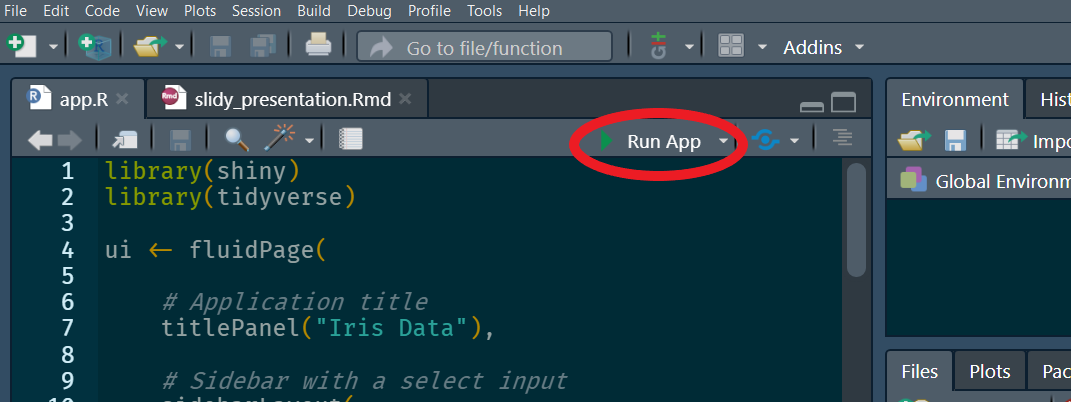02/12/2020

• shiny
• tidyverse
• DT

# Shiny Cheatsheet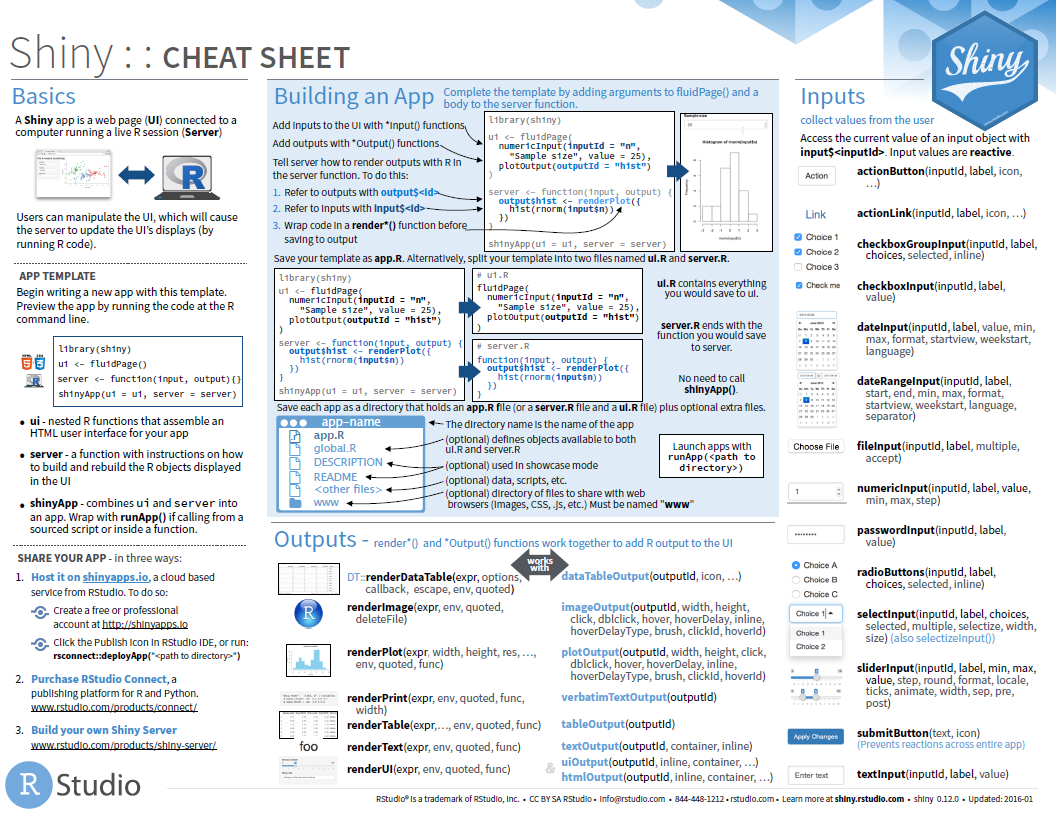# Example Shiny app

A live version of this app can be accessed here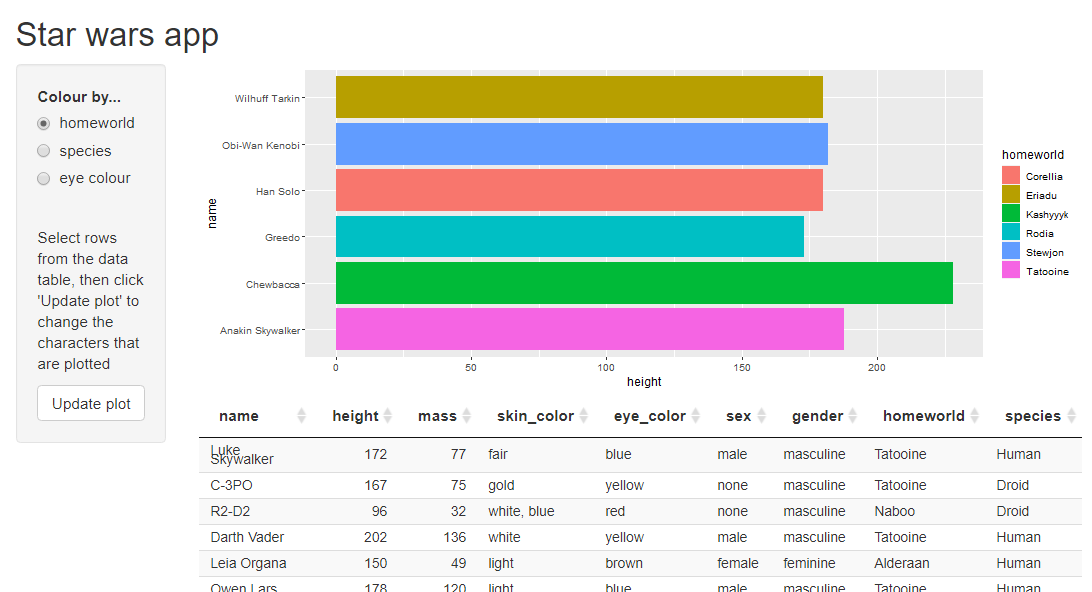# Another example app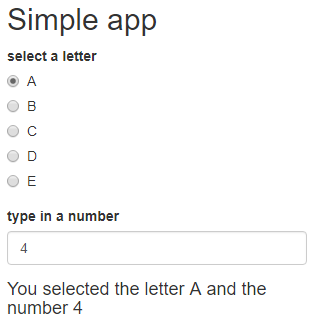# UI and Server

There are two parts to a Shiny application

• UI - the web page
• Server - the computer running the R session. This could be a personal computer or a remote machine.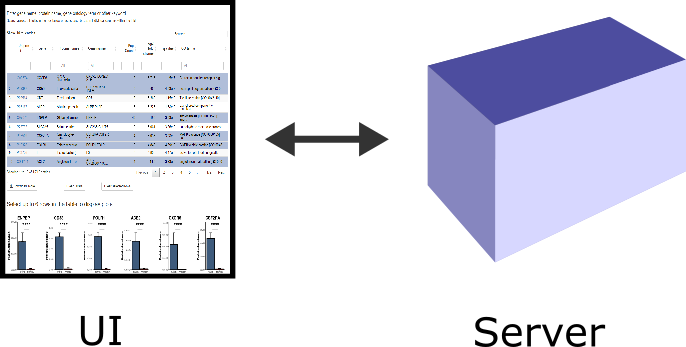# Skeleton Shiny code

``````library(shiny)

ui <- fluidPage(

)

server <- function(input, output, session) {

}

shinyApp(ui, server)``````

# Simple app code``````library(shiny)

ui <- fluidPage(

titlePanel("Simple app"),

label    = "select a letter",
choices  = LETTERS[1:5]),
numericInput(inputId = "number_choice",
label   = "type in a number",
value   = 4),
textOutput(outputId = "message")
)

server <- function(input, output) {

output\$message <- renderText({
paste0("You selected the letter ", input\$letter_choice,
" and the number ", input\$number_choice)
})
}

shinyApp(ui, server)``````

# UI code``````ui <- fluidPage(

titlePanel("Simple app"),

label    = "select a letter",
choices  = LETTERS[1:5]),

numericInput(inputId = "number_choice",
label   = "type in a number",
value   = 4),

textOutput(outputId = "message")
)``````

# Inputs

• Inputs are defined in the UI code.
• All inputs require a unique inputId. This gets added to the input object.
• The input object is a special type of named list (a reactiveValues object).
• The input object is passed to the server function and the values of the inputs can be accessed and used in the server code.
• The input object is read-only
``````ui <- fluidPage(
radioButtons(inputId = "letter_choice", label = "choose a letter", ...),
numericInput(inputId = "number_choice", label = "", ...)
)
server <- function(input, output) {
...
}  ``````

# Inputs

``````ui <- fluidPage(
numericInput(inputId = "number_choice", ...)
)
server <- function(input, output) {
...
}  ``````

The input object is a special type of named list.
From within the server code, the values of the input object can be accessed in the same way as list values.

``input\$letter_choice``
``"B"``

``input[["letter_choice"]]``
``"B"``
``input\$number_choice``
``4``

``input[["number_choice"]]``
``4``

# Server code

## Accessing and using the input values

``input\$letter_choice``
``"B"``
``input\$number_choice``
``4``

``````server <- function(input, output {

output\$message <- renderText({

paste0("You selected the letter ", input\$letter_choice,
" and the number ", input\$number_choice)
})
}``````

# Outputs

``````ui <- fluidPage(
...,
textOutput(outputId = "message")
)``````
• Plots/tables/text that can change according to user actions
• Initiated in the UI code and must contain a unique ID
• UI code functions as a placeholder
• Similar to the input object, output is list-like object named according to the output ID
• Input is read only, output is used for sending output

# Output in the simple app

Output function in UI code pairs with a render function in the server code.
Within the render function are instructions telling Shiny what to show the user.

``````ui <- fluidPage(...,
textOutput(outputId = "message")
)``````
``````server <- function(input, output, session) {
output\$message <- renderText({
paste0("You selected the letter ", input\$letter_choice,
" and the number ", input\$number_choice)
})
}  ``````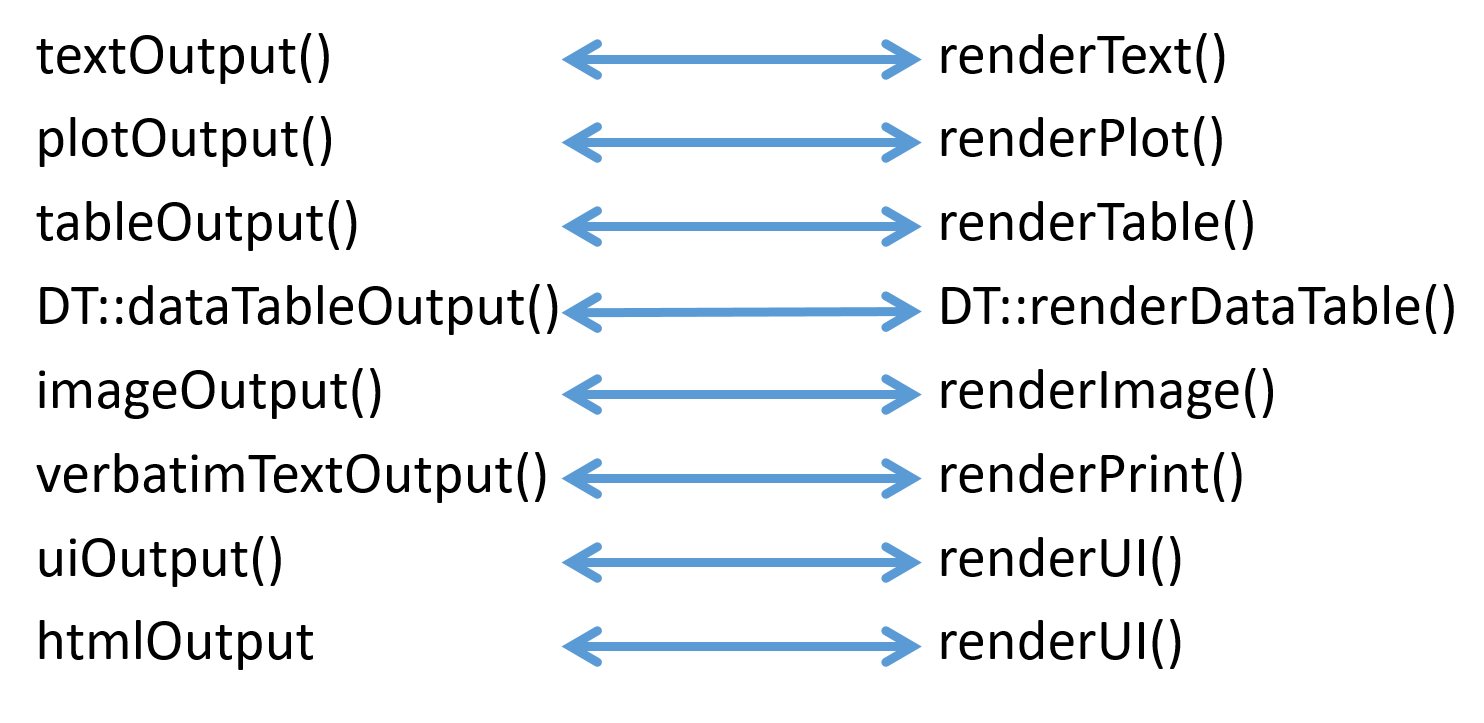# Recap``````library(shiny)
ui <- fluidPage(

titlePanel("Simple app"),
label    = "select a letter",
choices  = LETTERS[1:5]),
numericInput(inputId = "number_choice",
label   = "type in a number",
value   = 4),
textOutput(outputId = "message")
)

server <- function(input, output, session) {

output\$message <- renderText({

paste0("You selected the letter ", input\$letter_choice,
" and the number ", input\$number_choice)
})
}
shinyApp(ui, server)``````

# Running a shiny application